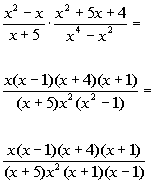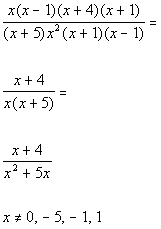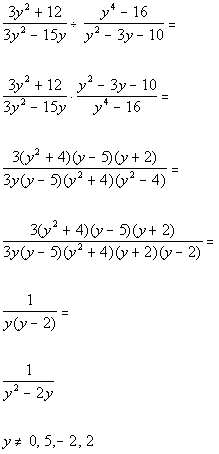TitleCollege Algebra
Answer/Discussion to Practice Problems
Tutorial 9: Multiplying and Dividing Rational ExpressionsAnswer/Discussion to 1aStep 1: Factor both the numerator and the denominator AND

 Step 2: Write as one fraction.*Factor the num. and den.

 In the numerator we factored a  GCF and a trinomial. In the denominator we factored a GCF and a difference of squares.

 Step 3: Simplify the rational expression. AND

 Step 4: Multiply any remaining factors in the numerator and/or denominator.*Simplify by div. out the common factors of  x, (x - 1) and (x + 1)       *Multiply the den. out     *Excluded values of the original den.

 Note that the values that would be excluded from the domain are 0, -5, -1, and 1.  Those are the values that makes the original denominator equal to 0.Answer/Discussion to 1bStep 1: Write as multiplication of the reciprocal AND  Step 2: Multiply the rational expressions as shown above.*Rewrite as mult. of reciprocal     *Factor the num. and den.           *Simplify by div. out the common factors of  3, (y^2 + 4), (y - 5), and (y + 2)   *Multiply the den. out       *Excluded values of the original den. of product

 In the numerator of the product we factored a GCF and a trinomial. In the denominator we factored a GCF  and a difference of squares.

 Note that even though all of the factors in the numerator were divided out there is still a 1 in there.  It is easy to think there there is "nothing" left and make the numerator disappear.  But when you divide a factor by itself there is actually a 1 there.  Just like 2/2 = 1 or 5/5 = 1. Note that the values that would be excluded from the domain are 0, 5, -2, and 2.  Those are the values that makes the original denominator of the product equal to 0.

Last revised on Dec. 15, 2009 by Kim Seward.
All contents copyright (C) 2002 - 2010, WTAMU and Kim Seward. All rights reserved.